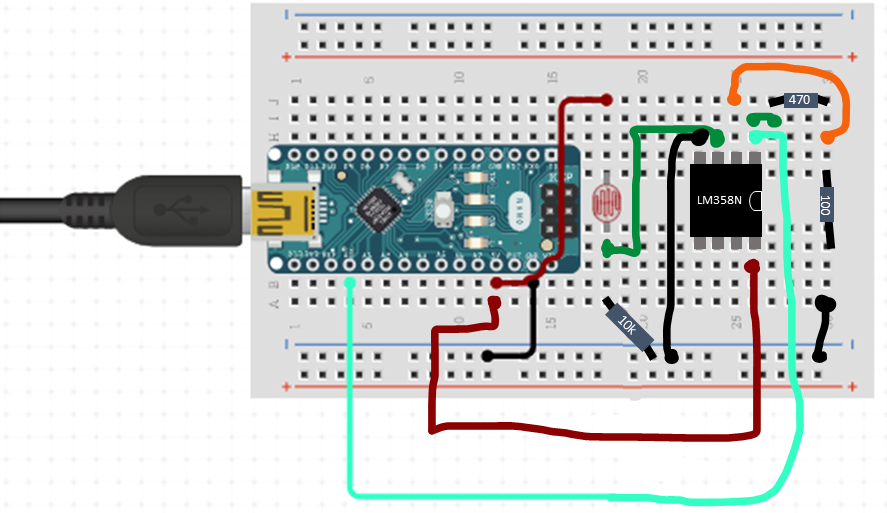# Arduino Lesson #8

Lesson Objective: Learn how to utilize an operational amplifier to increase the signal in a circuit. Observe the difference in analogRead as compared to digitalRead.

Materials Required:

• Wires
• Microprocessor with cable
• Resistors (100, 470 and 10k Ohms, or similar)
• Photoresistor (light dependent resistor, mine is a GL5539)
• Op-amp (mine is a Texas Instrument LM358N)
• The below Arduino sketch

Circuit diagram allowing a simple analogRead of photoresistor voltage drop:

Circuit diagram allowing analogRead of photoresistor voltage drop that has been amplified with an op-amp:This isn’t the best diagram as the circuito.io app did not have an op-amp in its wiring inventory and I had to hand-draw some of the wires.

Pin Configuration for LM358N Op-amp:

Arduino sketch for this lesson:

```const int sensorPin = A0 ; // select the input pin
int sensorValue = A0;  // Establishes that there is an integer that is called sensorValue. This block of memory on the chip now has a name.

void setup(void) {
Serial.begin(9600);
pinMode(sensorPin, INPUT);
}

void loop(void) {
sensorValue = analogRead(sensorPin); //tells the Arduino that the sensor value is an integer equal to the reading from A0
Serial.println(sensorValue); //tells the Arduino to print the value that was stored to the serial monitor
delay(1000);
}```